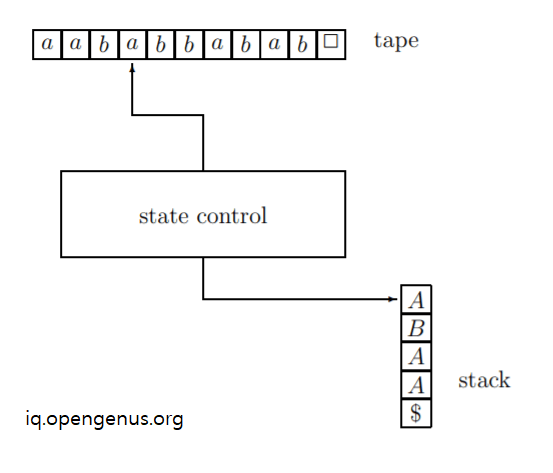# Pushdown Automata

Get FREE domain for 1st year and build your brand new siteIn this article, we have explained Pushdown Automata which is an important computational model in Theory of Computation and is used to accept Context Free Languages.

• Definition of Pushdown Automata
• Visualization of Pushdown Automata
• Pushdown Automata in Chomsky Hierarchy

Related topics you must learn about:

Let us get started with Pushdown Automata.

# Definition of Pushdown Automata

A Pushdown Automata is defined as a 5 tuple M = (Σ, Γ, Q, δ, q) where:

• Σ is the finite set of tape alphabet. Note this does not include blank symbol ✷.
• Γ: is a finite set of stack alphabet and this includes the special symbol \$.
• Q: is a finite set of states
• q is the start state and is a part of Q
• δ is the transition function. This is denoted as
``````δ: Q x (Σ ∪ { ✷ }) × Γ → Q × {N, R} × Γ ∗ .
``````

The transition function is the program of Pushdown Automata and it determines what a Pushdown Automata can do. One element of the transition function will be of the following form:

``````δ(r, a, A) = (r′, σ, w)
``````

This means:

• the Pushdown Automata is in state r
• the tape head of Pushdown Automata reads the input symbol a
• the top symbol on the stack of Pushdown Automata is A

With this, the Pushdown Automata makes the following changes:

• The Pushdown Automata moves to state r'
• the tape head of Pushdown Automata moves according to the value of σ: if σ = R, then we move one cell to the right and if σ = N, then we do not move.
• the top symbol A on the stack is removed and a new symbol w is inserted into the stack.

# Visualization of Pushdown Automata

This is how a Pushdown automata looks like:We have the following components:

• A tape
• A stack
• A State control

We take input from the tape and the state control determines the changes in the stack. The state control follows the rules in transition function.

In short, this computational model takes input in form of a tape and generates an output in the stack.

A Pushdown Automata accepts an input if both conditions hold true:

• It terminates on the input
• If a the time of termination, the tape head is on cell right of the last input cell and the current cell has a blank symbol.

Initial state of Pushdown Automata has:

• The tape head is on the leftmost input character.
• The state in the state control is q.
• The stack has only one element that is the special symbol \$.

For example of Pushdown Automata, go through this article where a Pushdown Automata for 0N 1N is presented.

# Pushdown Automata in Chomsky Hierarchy

This table gives you the idea of the different Languages in Theory of Computation and the position of Context Sensitive Language:

Type Language Automata Machine Power
Type 0 Recursively Enumerable Turing Machine Strongest
Type 1 Context Sensitive Linear Bounded Automaton Stronger
Type 2 Context Free Pushdown Automaton Strong
Type 3 Regular Finite State Automaton Weak

The above table is known as Chomsky Hierarchy and was first formed in 1956 by Noam Chomsky.

Therefore, we see a Context Free Language is accepted by a Pushdown Automata. Therefore, all possible strings generated by all possible Pushdown Automata is a set of all possible Context Free Language.

With this article at OpenGenus, you must have a strong idea of Pushdown Automata.